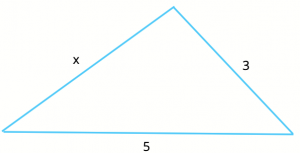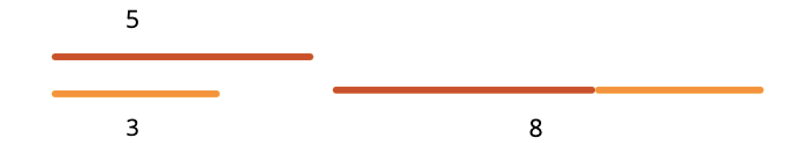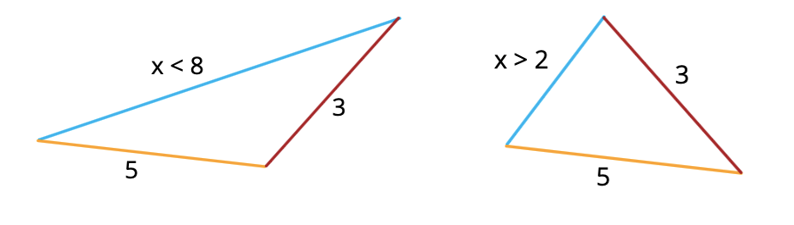One of the less-common but still need-to-know rules tested on the GMAT is the “triangle inequality” rule, which allows you to draw conclusions about the length of the third side of a triangle given information about the lengths of the other two sides.

Often times, this rule is presented in two parts, but I find it is easiest to condense it into one, simple part that concerns a sum and a difference. Here’s what I mean, and we’ll use a SCENARIO:

Suppose we have a triangle that has two sides of length 3 and 5:What can we say about the length of the third side? Of course, we can’t nail down a single definitive value for that length, but we can actually put a limit on its range. That range is simply the difference and the sum of the lengths of the other two sides, non-inclusive.

So, in this case, since the difference between the lengths of the other two sides is 2, and their sum is 8, we can say for sure that the third side of this triangle must have a length between 2 and 8, non-inclusive. [Algebraically, this reads as (5-3) < x < (5+3) OR 2 < x < 8.]

If you’d like to see that put into words:

**The length of any side of a triangle must be shorter than the sum of the other two side lengths and longer than the difference of the other two side lengths.**

It’s important to note that this works for any triangle. But why did we say non-inclusive? Well, let’s look at what would happen if we included the 8 in the above example. Imagine a “triangle” with lengths 3, 5, and 8. Can you see the problem? (Think about it before reading the next paragraph.)

Imagine a twig of length 3 inches and another of length 5 inches. How would you form a geometric figure of length 8 inches? You’d simply join the two twigs in a straight line to form a longer, single twig of 8 inches. It would be impossible to form a triangle with a side of 8 inches with the original two twigs.If you wanted to form a triangle with the twigs of 3 and 5, you’d have to “break” the longer twig of 8 inches and bend the two twigs at an angle for an opportunity to have a third side, guaranteed to be shorter than 8 inches:The same logic would hold for the other end of the range (we couldn’t have a triangle of 3, 5, and 2, as the only way to form a length of 5 from lengths of 2 and 3 would be to form a longer line segment of 5.)

Now that we’ve covered the basics, let’s dive into a few problems, starting with this Official Guide problem:

If k is an integer and 2 < k < 7, for how many different values of k is there a triangle with sides of lengths 2, 7, and k?
(A) one
(B) two
(C) three
(D) four
(E) five

As usual with the GMAT, it’s one thing to know the rule, but it’s another when you’re presented with a carefully worded question that tests your ability to pay close attention to detail. First, we are told that two of the lengths of the triangle are 2 and 7. What does that mean for the third side, given the triangle inequality rule? We know the third side must have a length between 5 (the difference between the two sides) and 9 (the sum of the two sides).

Here, you can actually use the answer choices to your advantage, at least to eliminate some answers. Notice that k is specified as an integer. How many integers do we know now are possible? Well, if k must be between 5 and 9 (and remember, it’s non-inclusive), the only options possibly available to us are 6, 7, and 8. That means a maximum of three possible values of k, thus eliminating answers D and E.

Since the GMAT is a time-intensive test, you might have to end up guessing now and then, so if you can strategically eliminate answers, it increases your chances of guessing correctly.

Now for this problem, there’s another condition given, namely that 2 < k < 7. We already determined that k must be 6, 7, or 8. However, of those numbers, only 6 fits in the given range 2 < k < 7. This means that 6 is the only legal value that fits for k. The correct answer is A.

## Note

It’s important to emphasize that the eliminate answers strategy is not a mandate. We’re simply presenting it as an option that works here because it is useful on many GMAT problems and should be explored and practiced as often as possible.

Check out the following links for our other articles on triangles and their properties:

By: Rich Zwelling, Apex GMAT Instructor
Date: 9th February, 2021